Question

# This program asks the user for the initial balance or principal (e.g. \$1234.56), the interest rate...

```This program asks the user for the initial balance or principal (e.g. \$1234.56),
the interest rate (as a percentage but without the % sign), number of years, and
how frequently compounded (supports the words "daily" for 365, "weekly" for 52,
"monthly" for 12, "quarterly" for 4, and "annually" for 1.```
```If then displays the total accrued amount (i.e. the principal plus the interest).

The output should look exactly like money.

Make sure you create each of the functions appropriately. All input() and
print() calls are in main() [do NOT use input() or print() in any ].

The file tests.py contains the unit tests that you will write for each function.
Make sure to open that file and complete the required tests.

EXAMPLE 1:
Principal: \$10000.00
Interest Rate: 3.875
Number of Years: 7.5
Frequency of Compounds (daily, weekly, monthly, quarterly, or annually): monthly
You will have accrued \$13366.37```

A simple formula can solve your problem. You need not have any complex functions in it. Since you did not specified to not use any formulae. I am just solving theses using the formula.

Note : Please mention programming language explicitly. Since you told "tests.py" as file name, I am assuming it as python and answering here.

Program :

#Input the principal

original_principal = int(input('Principal: '))

# Input the annual interest rate.

annual_interest = float(input('Interest Rate(%): '))
annual_interest = annual_interest / 100

# Input number of years account will earn interest.

total_years = float(input('Number of Years: '))

# Input times per year the interest is compounded.
c=0
while c==0:
compound = input("Frequency of Compounds (daily, weekly, monthly, quarterly, or annually):")
if compound=="annually":
c=1
elif compound=="monthly":
c=12
elif compound=="weekly":
c=52
elif compound=="daily":
c=365
elif compound=="quarterly":
c=4
else:
print("Please enter one of the option specified in brackets:")
# Calculate ending principle amount after earning
# interest for a specified amount of years.

ending_principal = (original_principal * (1 + annual_interest
/ c) ** (c * total_years)
)

# Display the ending principle amount.

print('At the end of ', total_years, 'years you will have \$',
format(ending_principal, '.2f'))

Outputs :

Test case 1 :

Here I am checking it for daily basis. Also I have written the code in such a way that. if user enters any other string for compounded frequency, it warns and prompts again.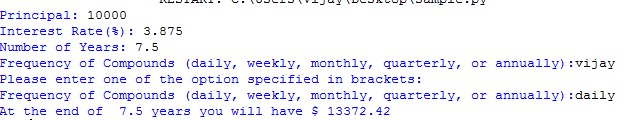Test Case 2:

This was the test case provided by you.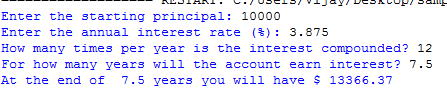Since the code is python , I am pasting the screen shot of the code so that you don't confuse with the indentation.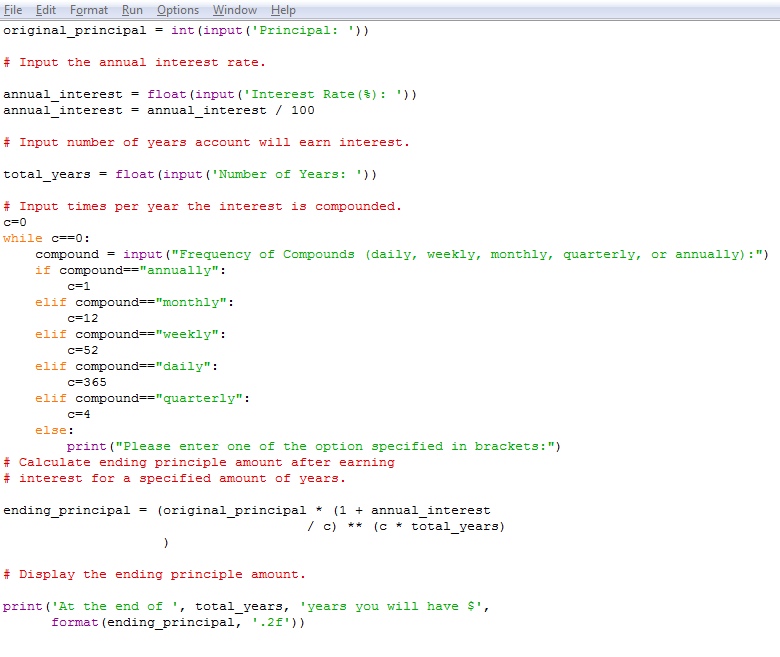#### Earn Coins

Coins can be redeemed for fabulous gifts.

Similar Homework Help Questions
• ### PROGRAMMING IN C. 1) The first program will compute compounded interest. Suppose you invest \$1000.00 at...

PROGRAMMING IN C. 1) The first program will compute compounded interest. Suppose you invest \$1000.00 at an interest rate of 2% per year. If the interest is computed at the end of each day, it is added to your account (i.e., the interest is compounded daily). Print what the account value will be at the end of each year for 20 years. Use data type double for money. For 365 days per year (ignore leap year), the daily interest rate...

• ### You take a 12-years fixed rate loan at 5.0 % annual interest rate with initial principal...

You take a 12-years fixed rate loan at 5.0 % annual interest rate with initial principal of \$400,000. The repayment is scheduled as quarterly instalments. (a) Solve for your quarterly payment. (6 marks) (b) Three years later, you decide the change to monthly instalment for the remaining period at the same interest rate. Solve for the monthly payment. (12 marks) (c) Calculate the total interest that you need to pay for the twelve years. (2 marks)

• ### You take a 12-years fixed rate loan at 5.0% annual interest rate with initial principal of \$400,000. The repayment is s...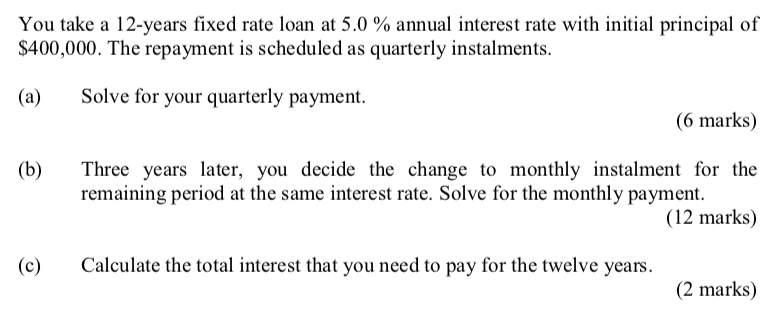You take a 12-years fixed rate loan at 5.0% annual interest rate with initial principal of \$400,000. The repayment is scheduled as quarterly instalments. (a) Solve for your quarterly payment. (6 marks) (b) Three years later, you decide the change to monthly instalment for the remaining period at the same interest rate. Solve for the monthly payment. (12 marks) c) Calculate the total interest that you need to pay for the twelve years. (2 marks)

• ### You need to save a total of \$7,500 in order to buy a new motorcycle. You...

You need to save a total of \$7,500 in order to buy a new motorcycle. You are starting with savings of \$4,000 but will make no additional contributions. The annual interest rate is 5%. In excel compute how long you would need to wait in order to reach your goal for interest that is compounded annually, semi-annually, quarterly, monthly, weekly, and daily. Which of the following accurately depicts this calculation? FV \$7,500 \$7,500 \$7,500 \$7,500 \$7,500 \$7,500 PV \$4,000 \$4,000...

• ### Wrote a program called ExceptionalDivide that asks the user for 2 integer values and displays the...

Wrote a program called ExceptionalDivide that asks the user for 2 integer values and displays the quotient of the first value divided by the second value. Make sure the your program reads in the two input values as ints. Your program must catch either of two exceptions that might arise and crash your program java.util.InputMismatchException //wrong data type inputted java.lang.ArithmeticException //divide by zero error Once you catch these exceptions, your program must print out a message explaining what happened and...

• ### Python assignment:  Write a program that asks for the user's age. Based on their response print "You...

Python assignment:  Write a program that asks for the user's age. Based on their response print "You can vote" (18 years old or older) or "You can't vote" and also whether or not he/she is a senior citizen (over 64). If senior citizen print "You're a Senior Citizen...you deserve two votes"!   Use the Idle editor and don't forget the .py extension. Good luck.          Your python program will be graded on the following:     Execution of the program gives the correct...

• ### ents due annually, on March 1. Each payment consists of \$20,000 principal plus one year's interest....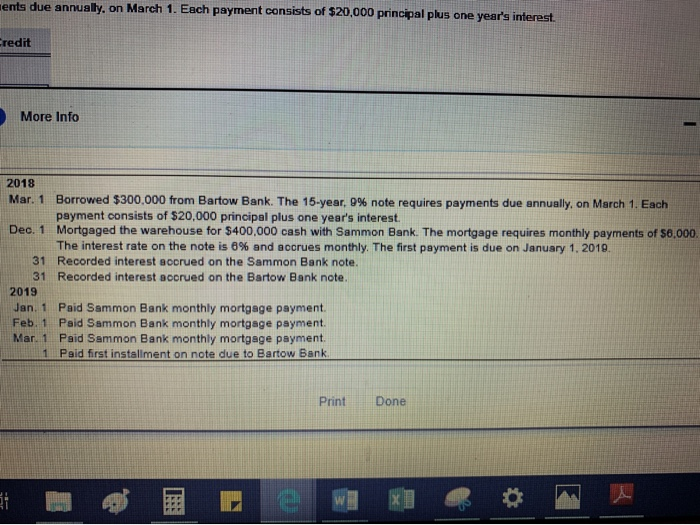ents due annually, on March 1. Each payment consists of \$20,000 principal plus one year's interest. redit More Info 2018 Mar. 1 Borrowed \$300,000 from Bartow Bank. The 15-year, 9% note requires payments due annually, on March 1. Each psyment consists of \$20,000 principal plus one year's interest Dec. 1 Mortgaged the warehouse for \$400,000 cash with Sammon Bank. The mortgage requires monthly payments of \$6,000. The interest rate on the note is 6 % and accrues monthly. The first...

• ### Project 1, Program Design 1. Write a C program replace.c that asks the user to enter...

Project 1, Program Design 1. Write a C program replace.c that asks the user to enter a three-digit integer and then replace each digit by the sum of that digit plus 6 modulus 10. If the integer entered is less than 100 or greater than 999, output an error message and abort the program. A sample input/output: Enter a three-digit number: 928 Output: 584 2. Write a C program convert.c that displays menus for converting length and calculates the result....

• ### You wish to buy a car for \$12,000 at a 5% annual interest rate, compounded monthly....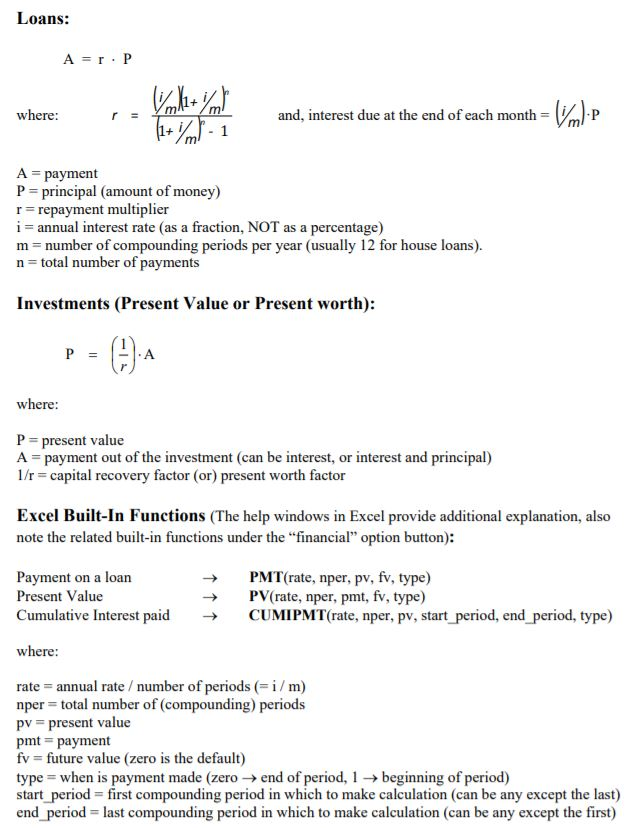You wish to buy a car for \$12,000 at a 5% annual interest rate, compounded monthly. The loan will be repaid in 5 years with monthly payments. What is your monthly payment (calculated with the equations on the next page)? Compare your answer to that obtained with the built in function, PMT. Be sure to label all cells appropriately. (There is no need to create a monthly payment table, simply use the equations on the next page.) Loans: where: and,...

• ### SOLVE USING C!!! Project objective: Conditional statements, loops, reading from file, user defined functions. **Submit source...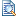SOLVE USING C!!! Project objective: Conditional statements, loops, reading from file, user defined functions. **Submit source code (LargeProg1.c) through Canvas One source code file(unformatted text) will be submitted Here is INCOMPLETE code to get started: LargeProg1.c The file name must match the assignment The code should be tested and run on a Microsoft compiler before it is uploaded onto Canvas The code must be submitted on time in order to receive credit (11:59PM on the due date) Late submissions will...